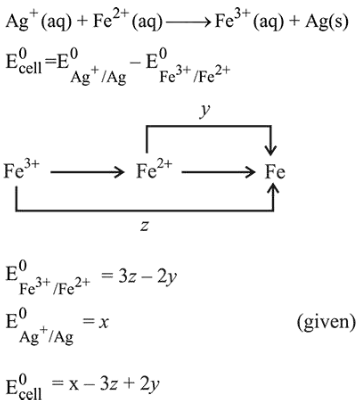# Calculate the standard cell potential (in V) of the cell in which following reaction takes place :Question:

Calculate the standard cell potential (in V) of the cell in which following reaction takes place :

$\mathrm{Fe}^{2+}(\mathrm{aq})+\mathrm{Ag}^{+}(\mathrm{aq}) \rightarrow \mathrm{Fe}^{3+}(\mathrm{aq})+\mathrm{Ag}(\mathrm{s})$

Given that

$\mathrm{E}_{\mathrm{Ag}^{+} / \mathrm{Ag}}^{0}=x \mathrm{~V}$

$\mathrm{E}_{\mathrm{Fe}^{2+} / \mathrm{Fe}}^{0}=y \mathrm{~V}$

$\mathrm{E}_{\mathrm{Fe}^{3+} / \mathrm{Fe}}^{0}=z \mathrm{~V}$

1. $x-z$

2. $x-y$

3. $x+2 y-3 z$

4. $x+y-z$

Correct Option: , 3

Solution: